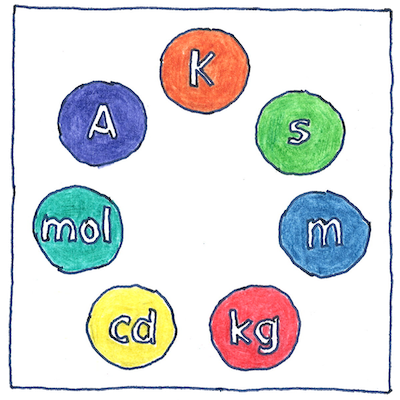# 1668,1799

## The book of science

Tom Sharp

 John Wilkins (and the French Revolution) metrology

## Metric system

• John Wilkins recognized
• that a standard system of measurement
• derived from something natural and measurable
• would reduce the difficulty
• of conducting trade between countries
• and proposed a system of tenths
• for magnitudes, weights, and values
• based on the length of a pendulum
• adjusted to swing every second
• such that subunits—tenths, hundredths,
• and multiples of ten, one hundred, and so on—
• and the standard units for volumes, weights, and values
• would be derived from the standard unit of length.
• A hundred years went by
• until the French revolted against abuse
• of arbitrary units of measurement
• by the privileged classes,
• and established, in 1799, metric units
• for length, area, and volume, to which were later added
• units for liquid, mass, and electrical amperage, voltage,
• resistance, power, capacitance, and charge.
• The French term metre was based on the Greek
• métron, which means measure.

## Eighths

• John Wilkins preferred a system of eighths
• because eight is more easily divided than ten
• but he deferred to the acceptance of counting based on ten,
• and the success of tenths in decimal arithmetic
• as Simon Stevin described in 1585.
• Wilkins also recognized the desirability
• of deriving the standard length
• from the longitude of the earth
• but admitted that this would be impractical.
• Christopher Wren suggested
• deriving the standard length from the pendulum,
• time being theoretically related to longitude
• and the revolution of the earth.

## Units

• John Wilkins dismissed the use of the barleycorn
• as the legal standard for length and weight in England.
• “The magnitude and weight of it
• may be so various in several times and places,
• as will render it incapable of serving for this purpose.”
• His standard length based on the pendulum
• was less than one percent shorter than a meter.
• The names of his proposed units
• were common English words;
• his standard length being called a standard.
• One tenth of a standard was a foot;
• one tenth of a foot was an inch;
• one hundred standards was a furlong;
• one thousand standards was a mile;
• and ten miles was a league.

## Conversion

• I’d walk one point six oh nine three four four kilometers
• in your size twenty three point eight centimeter shoes
• to feel your pain as a four on a scale of ten,
• but I have no idea how our city blocks
• add up to kilometers, and my size twenty seven point nine centimeter feet
• wouldn’t fit, literally, into your clogs,
• so my conversion will need to remain metaphorical.
• I hope to the nth degree that that’s good enough.

## Metric prefixing

• whole yotta going on
• zetta right there
• exactly
• peta pet
• tera infirma
• giga me a minute
• from alpha to omega
• kilo minute, kilo hour
• hecto, where was I?
• decimate
• a centi saved is a centi earned
• millipede
• microbrew
• nano nano
• pico de gallo
• the femto vote
• atto boy